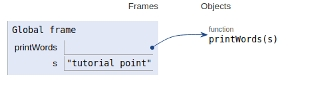# Python program to print even length words in a string

PythonServer Side ProgrammingProgramming

In this article, we will learn about the solution and approach to solve the given problem statement.

## Problem statement

Given a string we need to display all the words in the string with even length.

## Approach

• Split the input string using the split() function.

• Iterate over the words of a string using for a loop & Calculate the length of the word using len() function.

• If the length evaluates to be even, word gets displayed on the screen.

• Otherwise, no word appears on the screen.

Now let’s see the implementation given below −

## Example

def printWords(s):
# split the string
s = s.split(' ')
# iterate in words of string
for word in s:
# if length is even
if len(word)%2==0:
print(word)
# main
s = "tutorial point"
printWords(s)

## Output

tutorial

All the variables and functions are declared in the global scope as shown below −